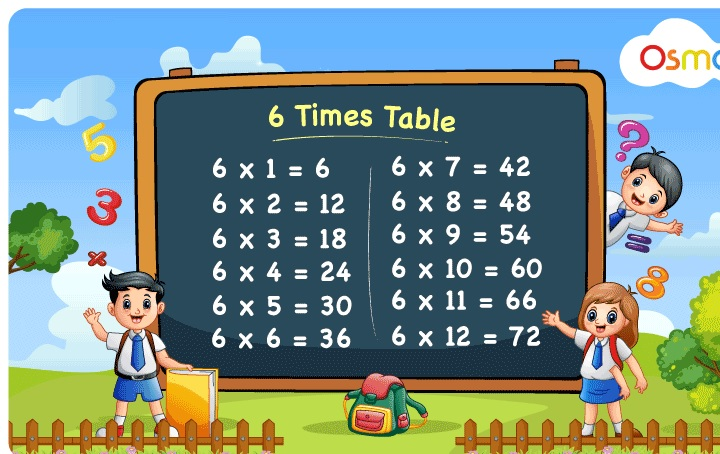Education

# Easy Tips to Learn Multiplication Table of 6 for KidsMultiplication tables are the building blocks of mathematics. Kids at the beginning learn tables from 1-10 so that they can solve the problems as per their understanding level. Once kids are acquainted with 1-5 tables, you can teach them tables of 6 in a creative way so that they can remember for a longer period of time. Acquiring knowledge on multiplication tables will enable children to solve any mathematical problem quickly. The best way to teach is by making them recite a table of 6 loudly for multiple times. This will make them remember the tables effortlessly.

Sometimes, kids need some easy tips which will help them to memorize the tables without putting much effort. Also, you need to make sure that the tips you incorporate in your lesson to teach 6 times table for kids should be easy and understandable. Anything which is difficult for kids to grasp would hamper their learning experience. Therefore, you need to come up with interesting activities and simple tips that can boost their confidence to learn more.

Kids from an early age should understand the importance of learning multiplication tables. They should be aware of the fact that the times table will help them solve arithmetic problems accurately. Most importantly, they will develop mathematical skills for better learning outcomes. Check out some of the tips and tricks to learn multiplication tables.

## Tips To Learn Table of 6 For Kids

Learning the multiplication table will enable kids to solve mathematical problems such as fractions, multiplication, division, percentage, etc. Kids should remember the tables in such a way that they are able to recall them instantly without putting much effort in thinking. A few tips to learn times table are mentioned below:

• When you multiply 6 with any even number, the last digit of the product will be the same. For example, 6 x 2= 12, 6 x 6= 36, 6 x 8= 48, 6 x 10= 60, 6 x 14= 84, etc.
• If you add 6 repeatedly to the numbers, you can find the answers for table 6. For example, 6 x 2= 12 , if you add 6 + 6= 12. Similarly, 6 x 8= 48, if you add 6+6+6+6+6+6+6+6 = 48.
• The product of table of 6 is double the table of 3. For example, 3 x 6= 18, if you add 18+18= 36, which is the product of a table of 6 x 6= 36.

### Table Of 6 Chart

Here is a table of 6 chart up to 20 for kids mentioned below:

 6 x 1= 6 6 x 2= 12 6 x 3= 18 6 x 4= 24 6 x 5= 30 6 x 6= 36 6 x 7= 42 6 x 8= 48 6 x 9= 54 6 x 10= 60 6 x 11= 66 6 x 12= 72 6 x 13= 78 6 x 14= 84 6 x 15= 90 6 x 16= 96 6 x 17= 102 6 x 18= 108 6 x 19= 114 6 x 20= 120

## Benefits Of Learning Times Table

Sometimes, kids might get intimidated to learn big numbers. Therefore, it is important to make them understand the use of multiplication tables in mathematics. Once they get acquainted with a 5 times table, you can move on to the higher numbers. Here are some of the advantages of learning multiplication tables mentioned below:

• Develops good mathematical skills.
• Enables children to solve mathematical problems accurately.
• Improves understanding of mathematical concepts.
• Develops higher retention and memory power.
• Improves academic performance with better results.

Excited
0
Happy
0
In Love
0
Not Sure
0
Silly
0

Education

### Popular B.Tech in Automobile Engineering Colleges In Ahmedabad

Engineering is a dynamic field, and you can choose from different verticals as per your ...
Education

### The Importance of Science Subject

School kids are very enthusiastic regarding new things being discovered, that leaves science a subject ...
Education

### 3 Career Skills You Can Develop During Quarantine

Due to the COVID-19 pandemic, people all over the world have been forced to stay ...

Education

### What Can You Expect From a Good School?

When it comes to taking care of children, there are many, many different aspects that ...
Education

### EXTREMOPHILES

What are Extremophiles? Extremophiles are organisms that thrive in extreme environments. Usually, these environments are ...
Education